﻿ 香港地区顾及高度改正的加权平均温度模型文章快速检索 高级检索
 大地测量与地球动力学2020, Vol. 40Issue (10): 1022-1026  DOI: 10.14075/j.jgg.2020.10.006### 引用本文CUI Jinye, MA Xiaping, LIU Xiaopeng. Weighted Mean Temperature Models with Vertical Adjustment for Hong Kong Region[J]. Journal of Geodesy and Geodynamics, 2020, 40(10): 1022-1026.### Foundation support

Open Fund of Key Laboratory for Modern Engineering Surveying of NASMG, No.TJES1810;Science and Technology Project of the Education Department of Shaanxi Province, No.18JK0513.

### Corresponding author

MA Xiaping, PhD, lecturer, majors in GNSS high-precise data processing, E-mail: celiang0321@163.com.

### 第一作者简介

CUI Jinye, teaching assistant, majors in modeling of tropospheric delay and precise positioning, E-mail: 475327506@qq.com.

### 文章历史

1. 吕梁学院矿业工程系, 山西省吕梁市学院路1号, 033001;
2. 西安科技大学测绘科学与技术学院, 西安市雁塔中路58号, 710054;
3. 中国水利水电第十二工程局有限公司, 杭州市环城北路141号, 310004

1 数据源与加权平均温度确定

 ${T_m} = \frac{{\int {\frac{e}{T}{\rm{d}}z} }}{{\int {\frac{e}{{{T^2}}}{\rm{d}}z} }} \approx \frac{{\sum\nolimits\limits_{i = 1}^N {\frac{{{e_i}}}{{{T_i}}}\Delta {h_i}} }}{{\sum\nolimits\limits_{i = 1}^N {\frac{{{e_i}}}{{T_i^2}}\Delta {h_i}} }}$ (1)

 $e = 6.112\exp \left[ {\frac{{17.67(T - 273.15)}}{{T - 29.65}}} \right]\frac{{{R_H}}}{{100}}$ (2)

2 顾及高度改正的Tm模型 2.1 香港地区Tm的垂直梯度

GNSS测站高度与模型参考高度的差异会影响Tm的精度，进而影响地基GNSS水汽获取的精度，因此精确的高度改正是Tm建模需要考虑的因素之一。Zhang等采用ERA-Interim大气再分析资料基于最小二乘方法确定了中国区域Tm的垂直递减率，发现其与高度呈线性关系。为了探索香港地区Tm与测站高度的关系，本文根据Zhang等的方法，利用Kings Park站2012~2017年探空数据，计算每个历元下的Tm高度递减率，结果见图 1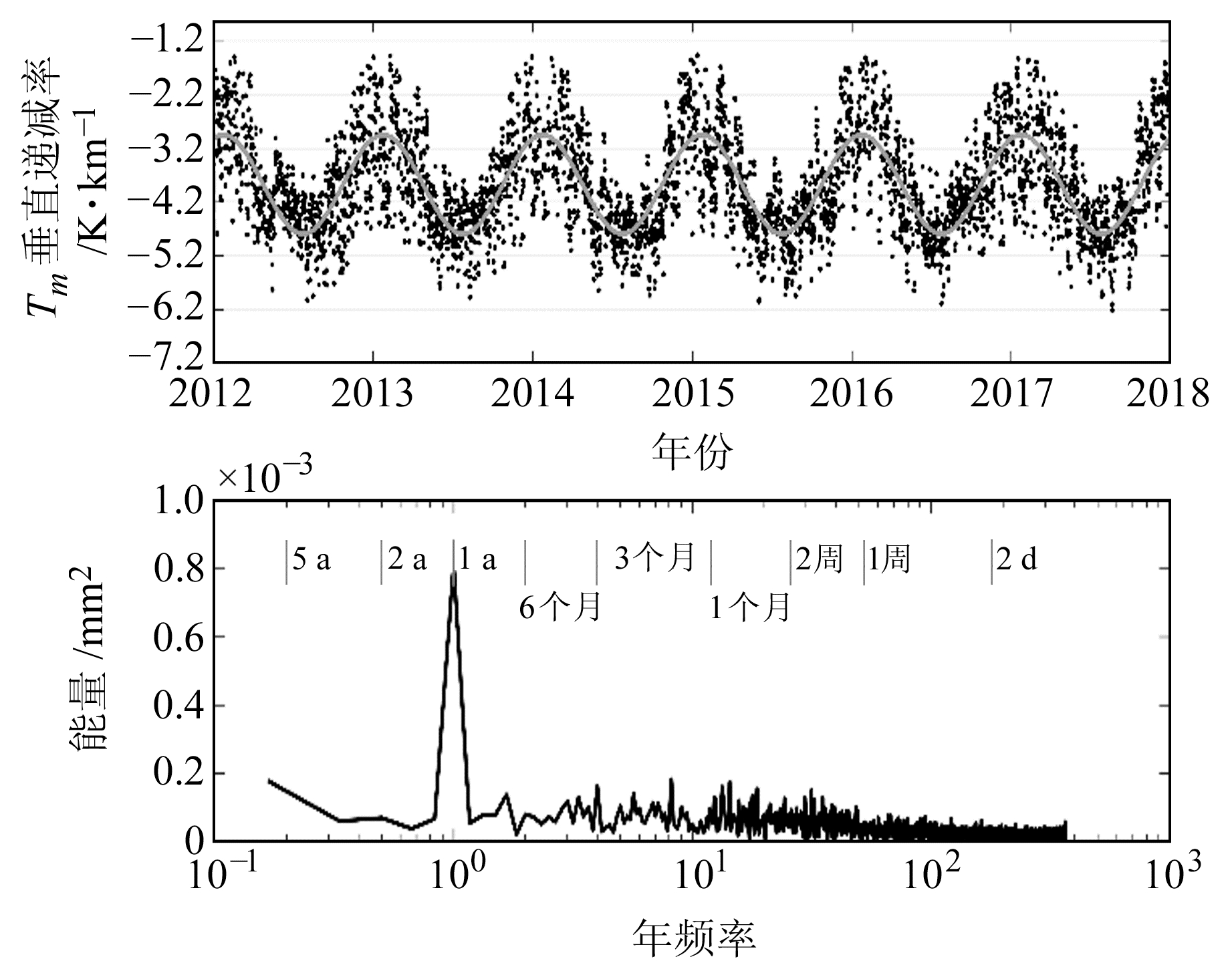图 1 Tm垂直递减率的时间序列和功率谱 Fig. 1 Time series and power spectrum of the vertical lapse rate in Tm

2.2 模型构建

 $\begin{array}{c} T_m^{{\rm{gnss}}} = T_m^{{\rm{ref}}} + \gamma ({h_{{\rm{gnss}}}} - {h_{{\rm{ref}}}})\\ = T_m^{{\rm{ref}}} + ({\gamma _1} + {\gamma _2}\cos (2\pi \frac{{{\rm{doy}}}}{{365.25}}) + \\ {\gamma _3}\sin (2\pi \frac{{{\rm{doy}}}}{{365.25}}))({h_{{\rm{gnss}}}} - {h_{{\rm{ref}}}}) \end{array}$ (3)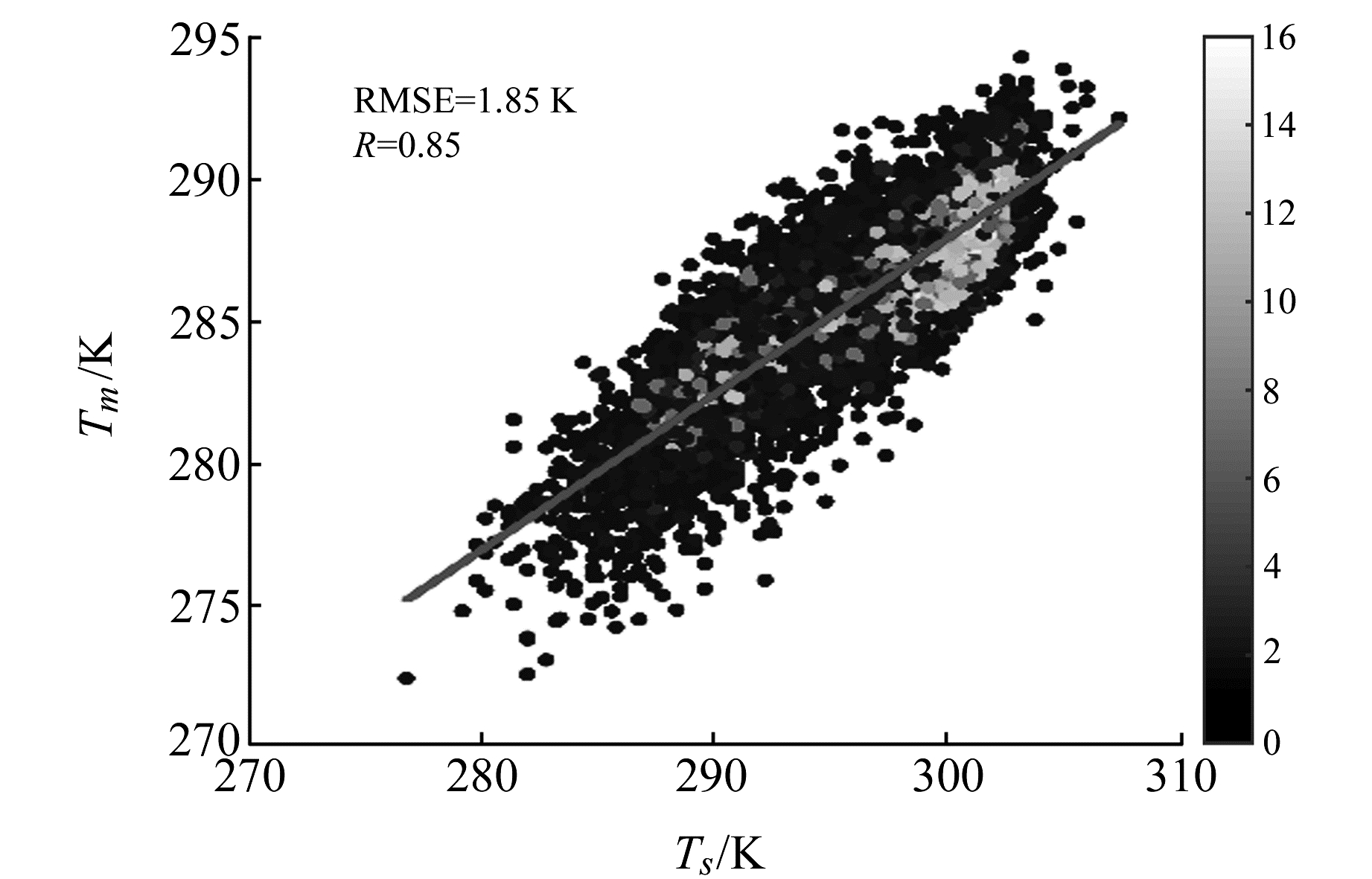图 2 Tm与地表温度的散点图 Fig. 2 Scatter of Tm and surface temperature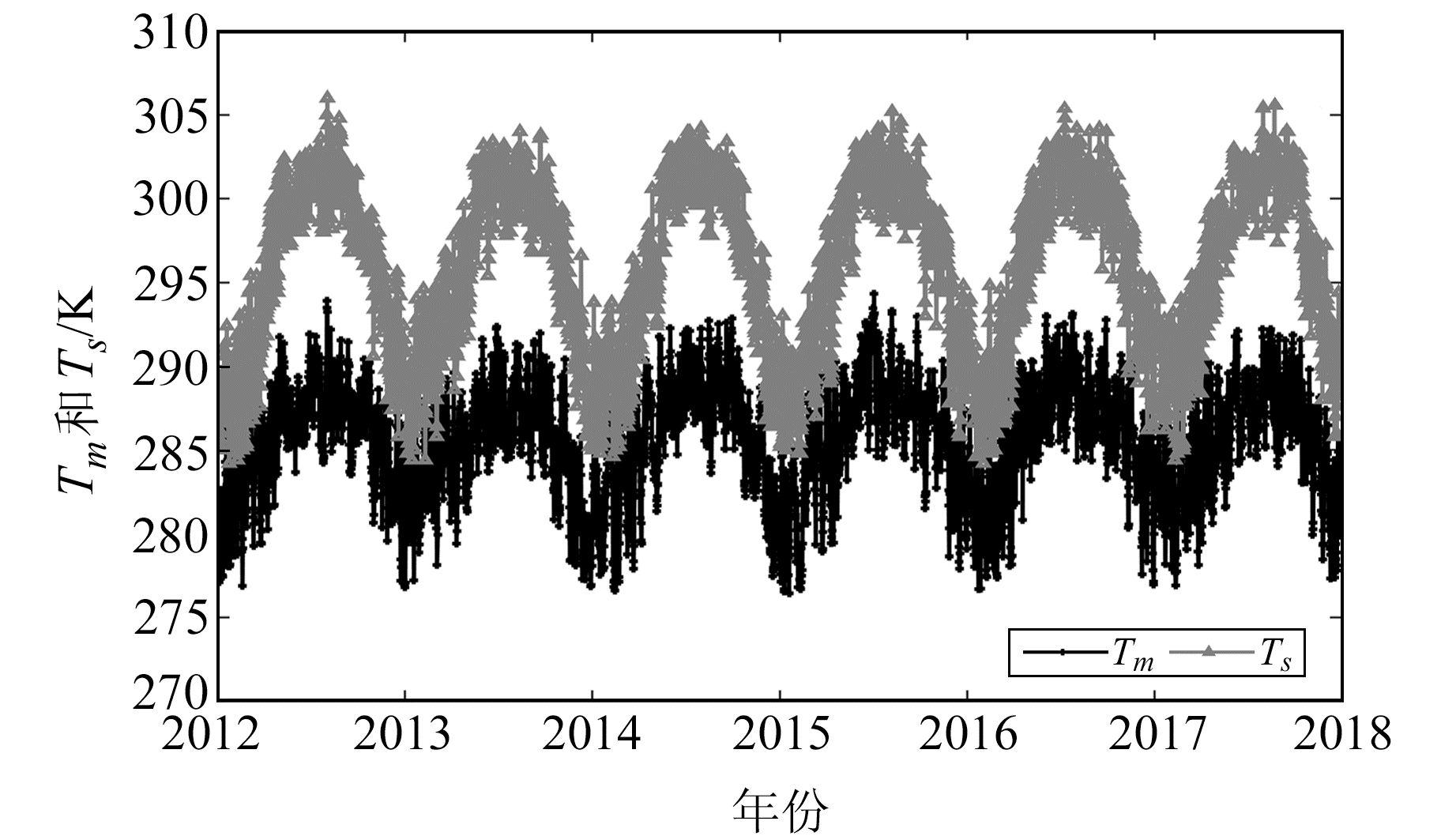图 3 Tm和Ts的时间序列 Fig. 3 Time series of Tm and Ts at reference height
 $\begin{array}{c} T_m^{{\rm{ref}}} = {\alpha _1} + {\alpha _2}T_s^{{\rm{ref}}} + {\alpha _3}\cos (2\pi \frac{{{\rm{doy}}}}{{365.25}}) + \\ {\alpha _4}\sin (2\pi \frac{{{\rm{doy}}}}{{365.25}}) + {\alpha _5}\cos (4\pi \frac{{{\rm{doy}}}}{{365.25}}) + \\ {\alpha _6}\sin (4\pi \frac{{{\rm{doy}}}}{{365.25}}) \end{array}$ (4)

Ts at reference height GNSS跟踪站主要用于定位、导航、授时及地球物理研究等，大多数没有配备共址的气象传感器，导致依赖气象参数的Tm模型很难应用于实时或近实时地基GNSS水汽的探测。因此，本文同时建立香港地区不依赖气象参数的Tm模型，以更好地服务于该地区暴雨、强对流等极端天气的水汽监测。图 3为参考高度TmTs的时间序列，可以看出，Tm具有明显的季节性周期。因此，这里采用Yao等的建模思路来拟合Tmref，建立不依赖气象参数的模型Tm_hk2。拟合公式如下：

 $\begin{array}{c} T_m^{{\rm{ref}}} = {\beta _1} + {\beta _{\rm{2}}}\cos (2\pi \frac{{{\rm{doy}}}}{{365.25}}) + \\ {\beta _{\rm{3}}}\sin (2\pi \frac{{{\rm{doy}}}}{{365.25}}) + {\beta _4}\cos (4\pi \frac{{{\rm{doy}}}}{{365.25}}) + \\ {\beta _5}\sin (4\pi \frac{{{\rm{doy}}}}{{365.25}}) \end{array}$ (5)表 1 Tm_hk1和Tm_hk2模型的系数 Tab. 1 Coefficients of Tm_hk1 and Tm_hk2 models
3 Tm模型在香港地区的精度和适用性分析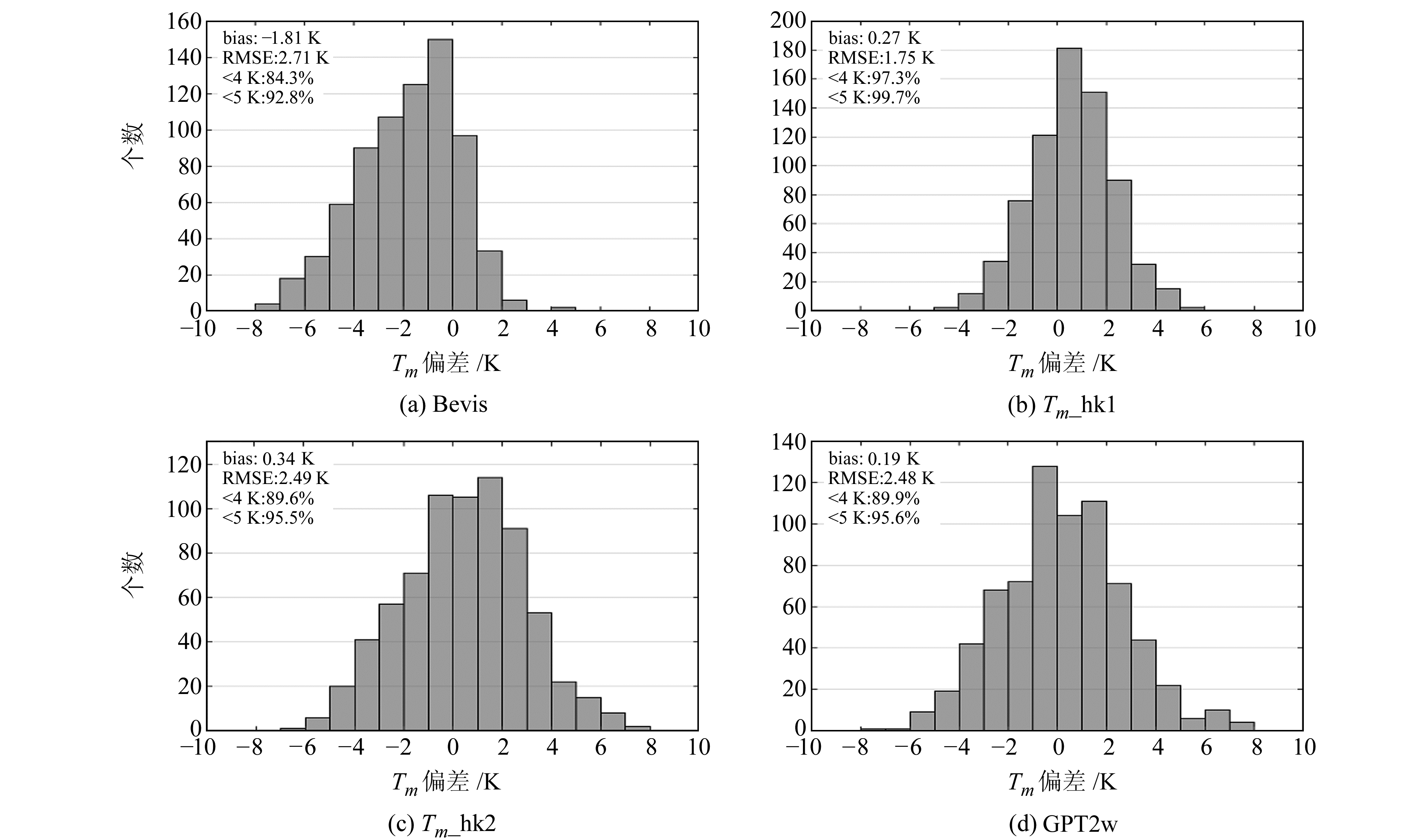图 4 4种Tm模型值偏差分布直方图 Fig. 4 Histogram of biases of Tm values for the four Tm models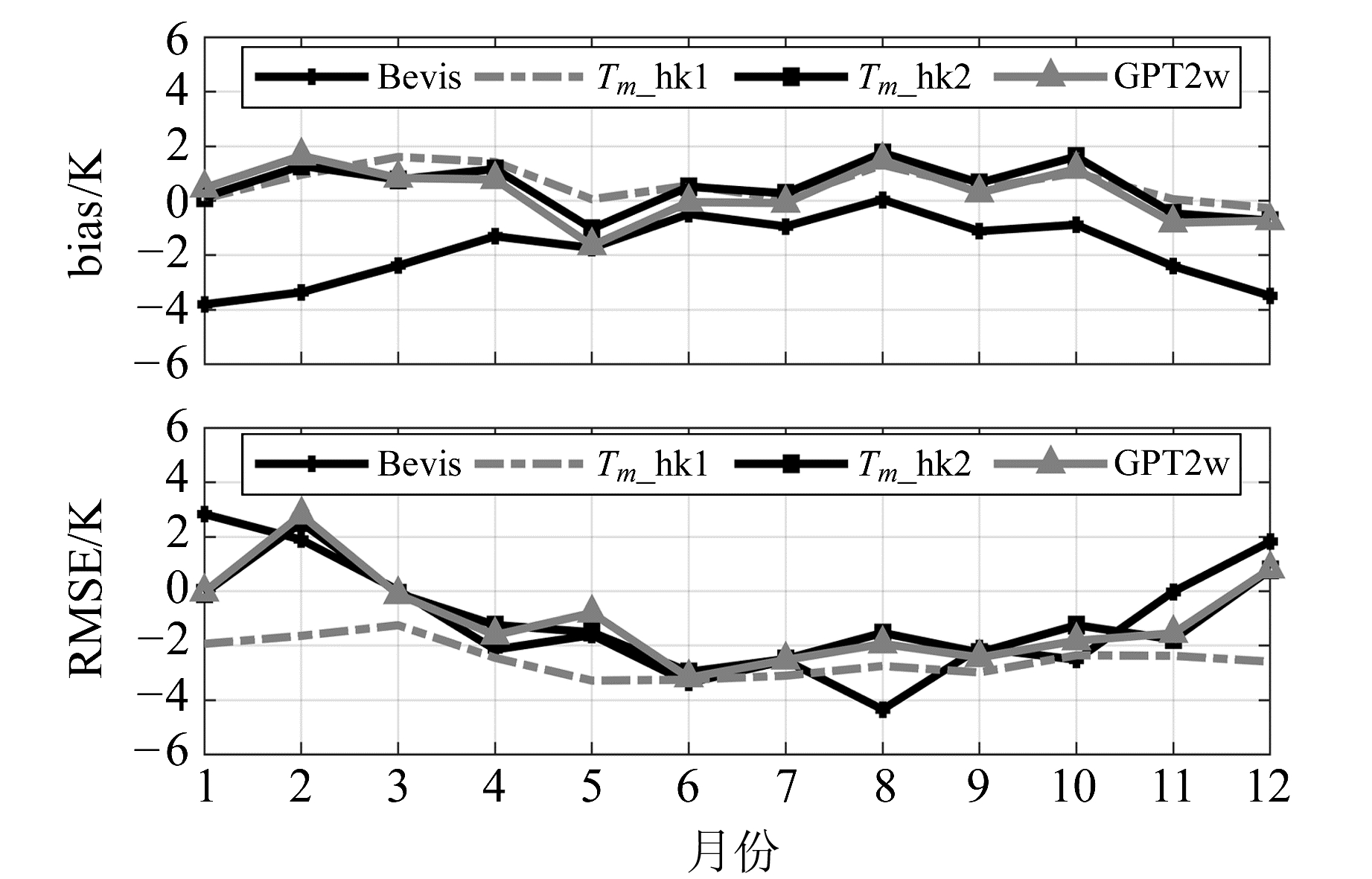图 5 4种模型Tm值的月平均偏差和均方根误差 Fig. 5 Monthly biases and RMS errors of Tm values for the four models表 2 4种Tm模型在不同季节的精度统计 Tab. 2 Statistics of precision of the four Tm models during seasons
4 结语

  Yao Y B, Zhang B, Xu C Q, et al. Improved One/Multi-Parameter Models that Consider Seasonal and Geographic Variations for Estimating Weighted Mean Temperature in Ground-Based GPS Meteorology[J]. Journal of Geodesy, 2014, 88(3): 273-282 DOI:10.1007/s00190-013-0684-6 (0)  Yao Y B, Xu C Q, Zhang B, et al. GTm-Ⅲ: A New Global Empirical Model for Mapping Zenith Wet Delays onto Precipitable Water Vapour[J]. Geophysical Journal International, 2014, 197(1): 202-212 DOI:10.1093/gji/ggu008 (0)  Zhang H X, Yuan Y B, Li W, et al. GPS PPP-Derived Precipitable Water Vapor Retrieval Based on Tm/Ps from Multiple Sources of Meteorological Data Sets in China[J]. Journal of Geophysical Research: Atmospheres, 2017, 122(8): 4165-4183 DOI:10.1002/2016JD026000 (0)  Ross R J, Rosenfeld S. Estimating Mean Weighted Temperature of the Atmosphere for Global Positioning System Applications[J]. Journal of Geophysical Research: Atmospheres, 1997, 102(D18): 21719-21730 DOI:10.1029/97JD01808 (0)  李建国, 毛节泰, 李成才, 等. 使用全球定位系统遥感水汽分布原理和中国东部地区加权"平均温度"的回归分析[J]. 气象学报, 1999, 57(3): 283-292 (Li Jianguo, Mao Jietai, Li Chengcai, et al. The Approach to Remote Sensing of Water Vapor Based on GPS and Linear Regression Tm in Eastern Region of China[J]. Acta Meteorological Sinica, 1999, 57(3): 283-292) (0)  王晓英, 戴仔强, 曹云昌, 等. 中国地区地基GPS加权平均温度Tm统计分析[J]. 武汉大学学报:信息科学版, 2011, 36(4): 412-416 (Wang Xiaoying, Dai Zaiqiang, Cao Yunchang, et al. Weighted Mean Temperature Tm Statistical Analysis in Ground-Based GPS in China[J]. Geomatics and Information Science of Wuhan University, 2011, 36(4): 412-416) (0)  王皓, 赵兴旺. 香港地区大气加权平均温度建模与研究[J]. 全球定位系统, 2019, 44(1): 119-124 (Wang Hao, Zhao Xingwang. Modeling and Research on Weighted Average Atmospheric Temperature in Hong Kong[J]. GNSS World of China, 2019, 44(1): 119-124) (0)  Yao Y B, Zhu S, Yue S Q. A Globally Applicable, Season-Specific Model for Estimating the Weighted Mean Temperature of the Atmosphere[J]. Journal of Geodesy, 2012, 86(12): 1125-1135 DOI:10.1007/s00190-012-0568-1 (0)  朱爽. 北京地区地基GPS加权平均温度计算本地化模型研究[J]. 测绘工程, 2014, 23(4): 28-32 (Zhu Shuang. Research of Local Model of Weighted Mean Temperature of Ground-Based GPS in Beijing[J]. Engineering of Surveying and Mapping, 2014, 23(4): 28-32) (0)  臧建飞, 彭秀英, 胡卓, 等. 顾及周期性误差修正的加权平均温度模型构建[J]. 测绘科学, 2019, 44(8): 149-160 (Zang Jianfei, Peng Xiuying, Hu Zhuo, et al. Building of Three Improved Weighted Mean Temperature Model Considering the Periodic Error Correction[J]. Science of Surveying and Mapping, 2019, 44(8): 149-160) (0)  Davis J L, Herring T A, Shapiro I I, et al. Geodesy by Radio Interferometry: Effects of Atmospheric Modeling Errors on Estimates of Baseline Length[J]. Radio Science, 1985, 20(6): 1593-1607 DOI:10.1029/RS020i006p01593 (0)  Wang X M, Zhang K F, Wu S Q, et al. Water Vapor-Weighted Mean Temperature and Its Impact on the Determination of Precipitable Water Vapor and Its Linear Trend[J]. Journal of Geophysical Research: Atmospheres, 2016, 121(2): 833-852 DOI:10.1002/2015JD024181 (0)
Weighted Mean Temperature Models with Vertical Adjustment for Hong Kong Region
CUI Jinye1MA Xiaping2LIU Xiaopeng3
1. Department of Mining Engineering, Lüliang University, 1 Xueyuan Road, Lüliang 033001, China;
2. College of Geomatics, Xi'an University of Science and Technology, 58 Mid-Yanta Road, Xi'an 710054, China;
3. Sinohydro Bureau 12 Co Ltd, 141 North-Huancheng Road, Hangzhou 310004, China
Abstract: Based on the iterative least square method, we develop two empirical Tm models for Hong Kong region: Tm_hk1 and Tm_hk2, with vertical adjustment using the radiosonde data of Kings Park station during the 2012-2017 period. The precision and reliability of the developed models, Bevis and global pressure temperature 2 wet(GPT2w), over Hong Kong region are evaluated using the sounding profiles throughout 2018. Results show that Tm_hk1, which requires the surface temperature at the station, can achieve a high precision with annual mean bias better than 0.3 K and the root mean square error(RMSE) within 1.8 K. Compared with the Bevis formula and GPT2w model, the accuracy of Tm_hk1 model increased by 35.4% and 29.7%, respectively. The Tm_hk2 model without the requirement of the meteorological parameter can achieve the same accuracy as the GPT2w model, and annual mean RMS error of both models are better than 2.5 K. Bevis formula has the worst accuracy(RMSE=2.7 K) and a large negative bias of -1.8 K. From the analysis, it can be found that the precision of Bevis, Tm_hk2, and GPT2w models show an obvious seasonal variation. The overall precision of the models during summer is higher(RMSE=1.3-2.2 K) than that during winter(RMSE=3.0-4.4 K). Furthermore, Tm_hk1 model performs the highest precision and applicability during all seasons, with the RMSE ranging from 1.4-2.4 K.
Key words: weighted mean temperature; modeling; Hong Kong region; precision analysis; atmospheric remote sensing